# Free online courseIntermediate Algebra by Leonard

5

(19)

## 54h38m of online video course

Quality content and selected for your learning.

## Exercises to train your knowledge

Many of the courses available have exercises to help with learning.

This course is free today and always will be, from start to finish you will pay nothing.

## Algebra

Study for free algebra, linear algebra, pre algebra and other with the free courses offered here. Our courses are the best free in all the webTeacher

##### Leonard
###### 6 Courses / 1743 students

This Channel is dedicated to quality mathematics education. It is absolutely FREE so Enjoy! Videos are organized in playlists and are course specific.

## Course content preview

There are no comments yet for this course.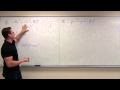Watch the 1st video of the course

## Course content2h17m

### Intermediate Algebra Lecture 6.1: Factoring the Greatest Common Factor (GCF)

Intermediate Algebra Lecture 6.1: Factoring the Greatest Common Factor (GCF)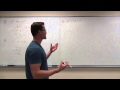0h54m

### Intermediate Algebra Lecture 6.2: Factoring Polynomials (Trinomials) With a = 1

Intermediate Algebra Lecture 6.2: Factoring Polynomials (Trinomials) With a = 1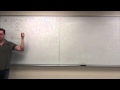0h53m

### Intermediate Algebra Lecture 6.4: Factoring Polynomials (Trinomials) in General

Intermediate Algebra Lecture 6.4: Factoring Polynomials (Trinomials) in General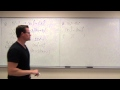1h39m

### Intermediate Algebra Lecture 6.5: Factoring Binomials (Difference of Squares/Cubes)

Intermediate Algebra Lecture 6.5: Factoring Binomials (Difference of Squares/Cubes and Sum of Cubes)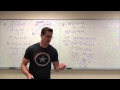1h23m

### Intermediate Algebra Lecture 6.6: Solving Equations by Factoring

Intermediate Algebra Lecture 6.6: Solving Equations by Factoring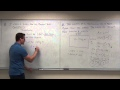1h05m

### Intermediate Algebra Lecture 6.7: Using Factoring to Solve Word/Application Problems

Intermediate Algebra Lecture 6.7: Using Factoring to Solve Word/Application Problems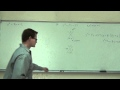2h28m

### Intermediate Algebra Lecture C.1: A BRIEF Review of Solving Equations and Factoring

Intermediate Algebra Lecture C.1: A BRIEF Review of Solving Equations and Factoring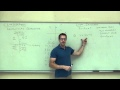0h38m

### Intermediate Algebra Lecture C.3: A BRIEF Review of Graphing

Intermediate Algebra Lecture C.3: A BRIEF Review of Graphing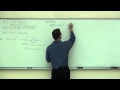0h39m

### Intermediate Algebra Lecture C.4: A BRIEF Review of Polynomial Mathematics

Intermediate Algebra Lecture C.4: A BRIEF Review of Addition, Subtraction, Multiplication, Division of Polynomials1h42m

### Intermediate Algebra Lecture 7.1: Defining and Simplifying Rational Expressions

Intermediate Algebra Lecture 7.1: Defining and Simplifying Rational Expressions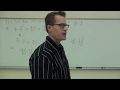1h08m

### Intermediate Algebra Lecture 7.2: How to Multiply and Divide Rational Expressions

Intermediate Algebra Lecture 7.2: How to Multiply and Divide Rational Expressions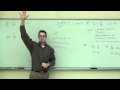1h10m

### Intermediate Algebra Lecture 7.3: Finding LCD and Equivalent Rational Expressions

Intermediate Algebra Lecture 7.3: Finding Lowest Common Denominator and Equivalent Rational Expressions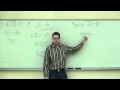1h16m

### Intermediate Algebra Lecture 7.4: Adding and Subtracting Rational Expressions

Intermediate Algebra Lecture 7.4: Adding and Subtracting Rational Expressions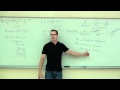0h57m

### Intermediate Algebra Lecture 7.5: Solving Rational Equations (Equations with Rational Expressions)

Intermediate Algebra Lecture 7.5: Solving Rational Equations (Equations with Rational Expressions)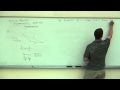0h49m

### Intermediate Algebra Lecture 7.6: Using Proportions and Rational Methods in Problem Solving

Intermediate Algebra Lecture 7.6: Using Proportions and Rational Methods in Problem Solving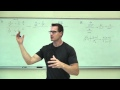0h40m

### Intermediate Algebra Lecture 7.7: Introducing and Simplifying Complex Fractions

Intermediate Algebra Lecture 7.7: Introducing and Simplifying Complex Fractions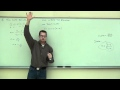1h41m

### Intermediate Algebra Lecture 8.1: Graphing Lines. Equations of Parallel and Perpendicular Lines

Intermediate Algebra Lecture 8.1: Graphing Linear Functions. Equations of Parallel and Perpendicular Lines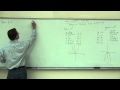0h46m

### Intermediate Algebra Lecture 8.2: An Introduction to Non-Linear Functions

Intermediate Algebra Lecture 8.2: An Introduction to Non-Linear Functions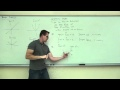1h21m

### Intermediate Algebra Lecture 8.3: Study of Piecewise Functions and Basic Translations of Graphs

Intermediate Algebra Lecture 8.3: Study of Piecewise Functions and Basic Translations of Graphs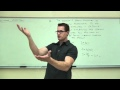0h46m

### Intermediate Algebra Lecture 8.4: An Introduction to Direct and Inverse Variation

Intermediate Algebra Lecture 8.4: An Introduction to Direct and Inverse Variation